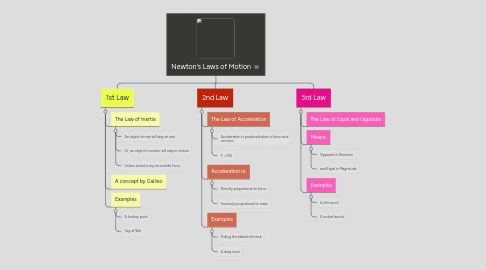# Newton's Laws of Motion

Get Started. It's FreeNewton's Laws of Motion## 1. 1st Law

### 1.1. The Law of Inertia

1.1.1. An object at rest will stay at rest

1.1.2. Or, an object in motion will stay in motion

1.1.3. Unless acted on by an outside force

### 1.3. Examples

1.3.1. A hockey puck

1.3.2. Tug of War

## 2. 3rd Law

### 2.2. Means:

2.2.1. Opposite in Direction

2.2.2. and Equal in Magnitude

### 2.3. Examples

2.3.1. A rifle recoil

2.3.2. A rocket launch

## 3. 2nd Law

### 3.1. The Law of Acceleration

3.1.1. Acceleration is produced when a force acts on mass

3.1.2. F = MA

### 3.2. Acceleration is:

3.2.1. Directly proportional to force

3.2.2. Inversely proportional to mass

### 3.3. Examples

3.3.1. Pulling the tablecloth trick

3.3.2. A drag racer